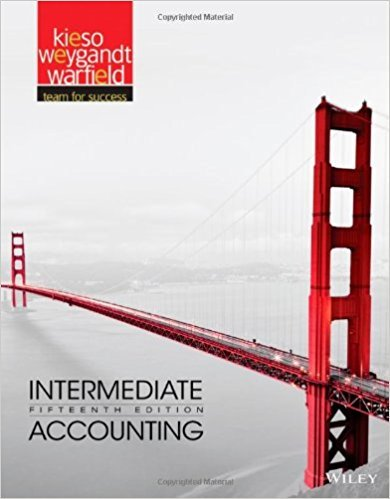×

×

# Solutions for Chapter 6: Accounting and the Time Value of Money## Full solutions for Intermediate Accounting | 15th Edition

ISBN: 9781118147290Solutions for Chapter 6: Accounting and the Time Value of Money

Solutions for Chapter 6
4 5 0 393 Reviews
16
1
##### ISBN: 9781118147290

Summary of Chapter 6: Accounting and the Time Value of Money

Since 19 problems in chapter 6: Accounting and the Time Value of Money have been answered, more than 39466 students have viewed full step-by-step solutions from this chapter. Chapter 6: Accounting and the Time Value of Money includes 19 full step-by-step solutions. Intermediate Accounting was written by and is associated to the ISBN: 9781118147290. This textbook survival guide was created for the textbook: Intermediate Accounting, edition: 15. This expansive textbook survival guide covers the following chapters and their solutions.

Key Business Terms and definitions covered in this textbook

the tendency for the mix of unobserved attributes to become undesirable from the standpoint of an uninformed party

• average revenue

total revenue divided by the quantity sold

• average tax rate

total taxes paid divided by total income

• Coase theorem

the proposition that if private parties can bargain without cost over the allocation of resources, they can solve the problem of externalities on their own

• common resources

goods that are rival in consumption but not excludable

• corrective tax

a tax designed to induce private decision makers to take account of the social costs that arise from a negative externality

• equality

the property of distributing economic prosperity uniformly among the members of society

• horizontal equity

the idea that taxpayers with similar abilities to pay taxes should pay the same amount

• income elasticity of demand

a measure of how much the quantity demanded of a good responds to a change in consumers’ income, computed as the percentage change in quantity demanded divided by the percentage change in income

• marginal product of labor

the increase in the amount of output from an additional unit of labor

the costs of changing prices

• microeconomics

the study of how households and firms make decisions and how they interact in markets

• nominal GDP

the production of goods and services valued at current prices

• price elasticity of supply

a measure of how much the quantity supplied of a good responds to a change in the price of that good, computed as the percentage change in quantity supplied divided by the percentage change in price

• quantity supplied

the amount of a good that sellers are willing and able to sell

• risk aversion

a dislike of uncertainty

• sacrifice ratio

the number of percentage points of annual output lost in the process of reducing inflation by 1 percentage point

• stock

a claim to partial ownership in a firm

• tariff

tax on goods produced abroad and sold domestically

• transaction costs

the costs that parties incur in the process of agreeing to and following through on a bargain